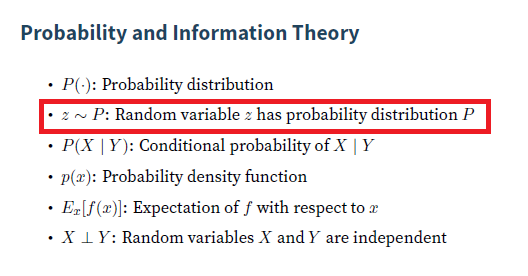# Notation

Hello,
Thanks for your brilliant book on deep learning.
I have a question and one suggestion.

Question:
As it’s shown in the image above (1st and 2nd lines), ‘z’ is a random variable, but why one is in small case and the other one is capital?

Suggestion:
I recommend you to use the word ‘given’ instead of vertical line as shown in 3nd line of the image, because you did not defined vertical line (|) yet.
Thanks

Hey @Aaron, thanks for the suggestion. The capital one should be the right one. Can I know where is the first line coming from? (I will go in to fix it!)

As the the vertical line, it is the math writing standard (and most people prefer concise than complicated, right?)

1 Like

Hi @goldpiggy , You are right.Thanks for your reply

Release: 0.16.6
Page: 14
Section: Probability and Information TheoryNotation, Set Theory, A∖B : set subtraction of B from A (contains only those elements of A that do not belong to B ). I don’t understand the “do not belong to B” part. For example, if A is a set containing “fish”, “dog” and B contains “fish”, “cat” is the result of A \ B simply “dog”? I.e., is it just removing items from A that both A and B have? If that is not correct then I don’t understand what “not belong to B” means. Thanks. All the rest of the notation I think I can grasp. Well done!

From what I understand. Set Subtraction is a way of changing a set by removing elements belonging to another set.
e.g. The set A − B or A \ B consists of elements that are in A but not in B. If A = {1,2,3} and B = {3,5}, then A − B = {1,2}.
So for your example A = {“Fish”, “Dog”} and B = {“Fish”}. So A \ B = {“Fish”}.

At bottom of page 14, it is written
“P(X | Y ): the conditional probability distribution of X given Y”

Notice that “P” is a probability measure and “X” and “Y” are random variables. For sets A and B, the notation P(A|B) is usual, however for random variables we do not typically write in this way, since random variables are measurable functions. The notation P(X \in A) actually represents P( X^{-1} (A) ) and P(X \in A | Y \in B) = P( X^{-1} (A) \cap Y^{-1}(B))/ P(Y^{-1}(B) ), provided that P(Y^{-1}(B))>0. Observe that X^{-1}(A) and Y^{-1}(B) are sets, but the objects X and Y are functions. Furthermore, the notation “X=x” represents the set X^{-1}( {x} )

The notation in the text is too informal and imprecise to represent conditional probabilities of random variables. Although, it is employed by some books and papers, formally speaking, this notation is wrong.

I recommend

1. “P(X=x | Y=y ): the conditional probability to the event where random variable X takes value x given that Y has taken value y”

Or

1. “P(X \in B | Y \in A): the conditional probability to the event where random variable X takes values in B given that Y has taken a value in A”

Best,
Alexandre Patriota,

A naive question here: is there a difference between “a tensor” and “a general tensor” which is denoted by X?

I believe it should be A \ B = {“Dog”}?
If the output was “Fish”, this represents an intersection.

1 Like

Thanks for that correction.I can’t find definitions of these two notations in this doc or from search engines.